# The MODEL Procedure

### Example 19.8 Nonlinear FIML Estimation

The data and model for this example were obtained from Bard (1974, pp. 133–138). The example is a two-equation econometric model used by Bodkin and Klein to fit U.S production data for the years 1909–1949. The model is the following: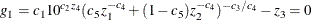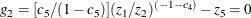where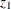is capital input,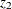is labor input,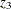is real output,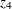is time in years with 1929 as year zero, and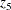is the ratio of price of capital services to wage scale. The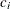’s are the unknown parameters.andare considered endogenous variables. A FIML estimation is performed by using the following statements:

```data bodkin;
input z1 z2 z3 z4 z5;
datalines;
1.33135 0.64629 0.4026 -20 0.24447
1.39235 0.66302 0.4084 -19 0.23454
1.41640 0.65272 0.4223 -18 0.23206

... more lines ...

```
```title1 "Nonlinear FIML Estimation";

proc model data=bodkin;
parms c1-c5;
endogenous z1 z2;
exogenous z3 z4 z5;

eq.g1 = c1 * 10 **(c2 * z4) * (c5*z1**(-c4)+
(1-c5)*z2**(-c4))**(-c3/c4) - z3;
eq.g2 = (c5/(1-c5))*(z1/z2)**(-1-c4) -z5;

fit g1 g2 / fiml ;
run;
```

When FIML estimation is selected, the log likelihood of the system is output as the objective value. The results of the estimation are shown in Output 19.8.1.

Output 19.8.1: FIML Estimation Results for U.S. Production Data

 Nonlinear FIML Estimation

The MODEL Procedure

Nonlinear FIML Summary of Residual Errors
Equation DF Model DF Error SSE MSE Root MSE R-Square Adj R-Sq
g1 4 37 0.0529 0.00143 0.0378
g2 1 40 0.0173 0.000431 0.0208

Nonlinear FIML Parameter Estimates
Parameter Estimate Approx Std Err t Value Approx
Pr > |t|
c1 0.58395 0.0218 26.76 <.0001
c2 0.005877 0.000673 8.74 <.0001
c3 1.3636 0.1148 11.87 <.0001
c4 0.473688 0.2699 1.75 0.0873
c5 0.446748 0.0596 7.49 <.0001

Number of Observations Statistics for System
Used 41 Log Likelihood 110.7773
Missing 0